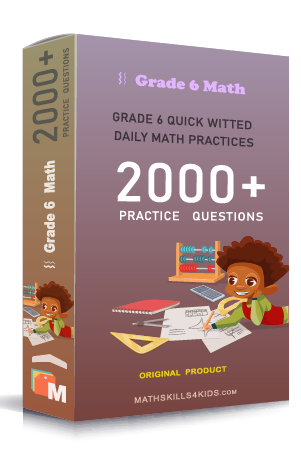# Grade 6 Multiplication worksheets with solutions

Subject
Math

Resource Type
Worksheets, Printables, Homeschool

Format
PDF (18.7 MB | 32 pages → Exercises + Solutions)

• ### -15% OFF Over \$100

Promo Code: TOPLA

After Purchase

\$2.85

This package allows you to practice the following skills

Multiplying whole numbers

Multiplying whole numbers word problems

Multiplying whole numbers with four or more digits

Multiplying numbers ending in zeroes

Multiplying numbers ending in zeroes word problems

Multiplying three or more numbers

Multiplying three or more numbers word problems

Estimate products

This product is a part of the Mega Pack
* Grade 6 quick-witted daily math practices *